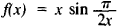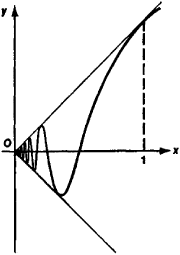length

(redirected from basinasal length)
Also found in: Dictionary, Thesaurus, Medical.

length

1. Prosody Phonetics the metrical quantity or temporal duration of a vowel or syllable
2. the distance from one end of a rectangular swimming bath to the other
3. Prosody the quality of a vowel, whether stressed or unstressed, that distinguishes it from another vowel of similar articulatory characteristics. Thus in English beat is of greater length than in English bit
4. Cricket the distance from the batsman at which the ball pitches
5. Bridge a holding of four or more cards in a suit
Collins Discovery Encyclopedia, 1st edition © HarperCollins Publishers 2005

Length

A one-dimensional extension in space. Length is one of the three fundamental physical quantities, the others being mass and time. It can be measured by comparison with an arbitrary standard; the specific one in most common usage is the international meter. In 1983, at the meeting of the Conférence Général des Poids et Mésures, the meter was redefined in terms of time and the speed of light: “The meter is the length of the path traveled by light in vacuum during a time interval of 1/299 792 458 of a second.” This definition defines the speed of light to be exactly 299 792 458 m/s, and defines the meter in terms of the most accurately known quantity, the second. See Light, Mass, Time

McGraw-Hill Concise Encyclopedia of Physics. © 2002 by The McGraw-Hill Companies, Inc.
The following article is from The Great Soviet Encyclopedia (1979). It might be outdated or ideologically biased.

Length

a numerical characteristic of the extent of curves. The concept of length is defined differently for different cases. (1) The length of a line segment is the distance between its end points, measured by some segment taken as a unit of length. (2) The length of a polygonal line is the sum of the lengths of its components. (3) The length of a simple arc is the limit of the lengths of polygonal lines inscribed in the arc, when the number of components increases indefinitely and the maximum length of the components tends to zero. (4) The length of a continuous curve consisting of a finite number of simple arcs is equal to the sum of the lengths of these arcs. For example, the circumference of a circle can be obtained as the limit of the perimeters of inscribed regular polygons when the number of their sides is doubled indefinitely; it is equal to 2πR where R is the radius of the circle. Any continuous curve has finite or infinite length. If its length is finite, then the curve is said to be rectifiable. The graph (see Figure 1) of the functionfor 0 <x ≤ 1 and f(x) = 0 when x = 0 is an example of a nonrectifiable curve; here the lengths of the inscribed polygonal lines increase beyond all bound as the length of the components tend to zero. If the equation of a plane curve has the form y =f(x) (a ^ x ^ b)m rectangular coordinates and the function f(x) has a continuous derivative f’(x), then the length of the curve is given by the integralThe length of a curve given in parametric form and the length of a space curve can be expressed in a similar manner.Figure 1

To calculate the length of a curve the mathematicians of antiquity essentially used lengths of polygonal lines and passage to the limit. For them, however, such passage to the limit was only a method for calculating the length of a curve and not for defining the concept of the length of a curve, since they apparently perceived the latter as one of the elementary mathematical concepts. The necessity of defining the length of a curve became clear only in the first half of the 19th century. A full elucidation of the problem was achieved by C. Jordan. In differential geometry the length of a curve is also defined on a surface or in an arbitrary Riemannian space.

REFERENCES

Lebesgue, H. Ob izmerenii velichin, 2nd ed. Moscow, 1960. (Translated from French.)
Fikhtengol’ts, G. M. Kurs differentsial’nogo i integral’nogo ischisleniya, 7th ed. vol. 2. Moscow, 1969.